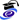# Leonard Peter BosPosition
Full Professor
MAT/08 - NUMERICAL ANALYSIS
Research sector (ERC)
PE1_17 - Numerical analysis

Office
Ca' Vignal 2,  Floor 2,  Room 8
Telephone
+39 045 802 7987
Fax
+39 045 802 7068
E-mail
leonardpeterbosunivrit#### Office Hours

Monday, Hours 3:30 PM - 5:30 PM,   Ca' Vignal 2, Floor 2, room 1

Curriculum
•Curriculum - (En, pdf)   (pdf, en, 150 KB, 14/01/19)
•Curriculum - (It, pdf)   (pdf, it, 150 KB, 14/01/19)

Len Bos si occupa di:
• punti (quasi) ottimali per l'interpolazione polinomiale nel caso di più di una variabile
• inugualianze di tipo Bernstein/Markov per polinomi di più di una variabile
• la teoria di pluripotenziale e le sue applicazioni
Le sue pubblicazioni si collocano prevalentemente sulle riviste internazionali dell'area dell'analisi numerica, ma non solo.

#### Modules

Modules running in the period selected: 41.
Click on the module to see the timetable and course details.

Course Name Total credits Online Teacher credits Modules offered by this teacher
Master's degree in Mathematics Data Fitting and Reconstruction (2019/2020)   6
Bachelor's degree in Applied Mathematics Numerical analysis II with laboratory (2019/2020)   6    (Teoria)
Bachelor's degree in Applied Mathematics Numerical analysis I with laboratory (2019/2020)   6    (Teoria)
Master's degree in Mathematics Numerical methods for mathematical finance (seminar course) (2019/2020)   6
Master's degree in Mathematics Advanced numerical analysis (2018/2019)   6
Bachelor's degree in Applied Mathematics Numerical analysis II with laboratory (2018/2019)   6    (Teoria)
Bachelor's degree in Applied Mathematics Numerical analysis I with laboratory (2018/2019)   6    (Teoria)
Master's degree in Mathematics Numerical methods for mathematical finance (seminar course) (2018/2019)   6
Master's degree in Mathematics Statistical methods for data analysis (2018/2019)   6(Statistical modelling)
Master's degree in Mathematics Advanced numerical analysis (2017/2018)   6
Bachelor's degree in Applied Mathematics Numerical analysis II with laboratory (2017/2018)   6
Bachelor's degree in Applied Mathematics Numerical analysis I with laboratory (2017/2018)   6Master's degree in Mathematics Numerical methods for mathematical finance (seminar course) (2017/2018)   6
Master's degree in Mathematics Advanced numerical analysis (2016/2017)   6
Bachelor's degree in Applied Mathematics Numerical analysis with laboratory (2016/2017)   12    (Teoria)
Master's degree in Mathematics Numerical methods for mathematical finance (seminar course) (2016/2017)   6Master's degree in Mathematics Advanced numerical analysis (2015/2016)   6
Bachelor's degree in Applied Mathematics Numerical analysis with laboratory (2015/2016)   12    (teoria)
Master's degree in Mathematics Numerical methods for mathematical finance (seminar course) (2015/2016)   6Master's degree in Mathematics Scientific computing (seminar course) (2015/2016)   6
Master's degree in Mathematics Advanced numerical analysis (2014/2015)   6
Master's degree in Mathematics Mathematical finance (2014/2015)   6    (Teoria 2)
Bachelor's degree in Applied Mathematics Numerical analysis with laboratory (2014/2015)   12    (Teoria)
Master's degree in Mathematics Numerical methods for mathematical finance (seminar course) (2014/2015)   6
Master's degree in Mathematics Advanced numerical analysis (2013/2014)   12    (Teoria)
Master's degree in Mathematics Mathematical finance (2013/2014)   6    (Teoria 2)
Bachelor's degree in Applied Mathematics Numerical analysis with laboratory (2013/2014)   12    (Teoria)
Master's degree in Mathematics Numerical methods for mathematical finance (seminar course) (2013/2014)   6
Master's degree in Mathematics Scientific computing (seminar course) (2013/2014)   6
Master's degree in Mathematics Advanced numerical analysis (2012/2013)   12    (Teoria)
Master's degree in Mathematics Approximation of scattered data (seminar course) (2012/2013)   6
Bachelor's degree in Computer Science Numerical Analysis (2012/2013)   6    (Teoria)
Bachelor's degree in Applied Mathematics Numerical analysis with laboratory (2012/2013)   12    (Teoria)
Master's degree in Mathematics Advanced numerical analysis (2011/2012)   12    (Teoria)
Bachelor's degree in Applied Mathematics Numerical analysis with laboratory (2011/2012)   12    (Teoria)
Master's degree in Mathematics L'approssimazione di dati sparsi (2011/2012)   6
Master's degree in Mathematics Advanced numerical analysis (2010/2011)   12    (Teoria)
Bachelor's degree in Applied Mathematics Numerical analysis with laboratory (2010/2011)   12    (Teoria)
Master's degree in Mathematics Metodi numerici avanzati per le equazioni differenziali (2010/2011)   6
Bachelor's degree in Applied Mathematics Numerical analysis with laboratory (2009/2010)   12(Teoria)
Bachelor's degree in Computer Science Probability and Statistics (2009/2010)   6    (Teoria)

#### Research groups

Mathematics - applications and modelling
Skills
Topic Description Research area
Numerical approximation We implement algorithms to calculate a numerical approximation of a complicated function, defined either directly by an explicit formula or procedure or else, for example, defined indirectly as the solution of a differential equation of some type. Mathematics - applications and modelling
Numerical analysis
Inequalities We study polynomial inequalities of Markov/Bernstein type for the derivatives of multivariate polynomials. Mathematics - applications and modelling
Inequalities For maximal function inequalities
Multivariate Polynomial Interpolation We study optimal points and their asymptotic distribution for polynomial interpolation on a compact set in R^n Mathematics - applications and modelling
Approximations and expansions
Pluripotential theory A function defined on C^n is said to be plurisubharmonic if restricted to every complex line it is a subharmonic function of one variable. Pluripodtential Theory is the study of such functions and is, in particular, the correct theory for the study of multivariate polynomials. Mathematics - applications and modelling
Pluripotential theory
Projects
Title Starting date
Approssimazione multivariata con basi polinomiali e radiali 2/29/12
Interpolazione multivariata con polinomi, RBF e altre basi e applicazioni (PRIN 2009) 7/15/11
Near Optimal Points for Multivariate Interpolation 1/1/10